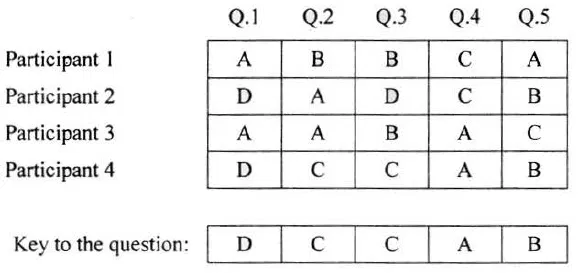Example Programs

Program 

The result of a quiz competition is to be prepared as follows:

The quiz has five questions with four multiple choices (A, B, C, D), with each question carrying 1 mark for the correct answer. Design a program to accept the number of participants N such that N must be greater than 3 and less than 11. Create a double dimensional array of size (Nx5) to store the answers of each participant row-wise.

Calculate the marks for each participant by matching the correct answer stored in a single dimensional array of size 5. Display the scores for each participant and also the participant(s) having the highest score.

Example: If the value of N = 4, then the array would be:Test your program with the sample data and some random data:

Example 1

INPUT : N = 5

Participant 1 D A B C C

Participant 2 A A D C B

Participant 3 B A C D B

Participant 4 D A D C B

Participant 5 B C A D D

Key: B C D A A

OUTPUT : Scores :

Participant 1 D A B C C

Participant 1 = 0

Participant 2 = 1

Participant 3 = 1

Participant 4 = 1

Participant 5 = 2

Highest score: Participant 5

Example 2

INPUT : N = 4

Participant 1 A C C B D

Participant 2 B C A A C

Participant 3 B C B A A

Participant 4 C C D D B

Key: A C D B B

OUTPUT : Scores :

Participant 1 = 3

Participant 2 = 1

Participant 3 = 1

Participant 4 = 3

Highest score:

Participant 1

Participant 4

Example 3

INPUT : N = 12

OUTPUT : INPUT SIZE OUT OF RANGE.